# Plateau problem

The problem of finding a minimal surface with a given boundary. The problem was first formulated by J.L. Lagrange (1760), who reduced it for the class of surfaces of the form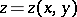to the solution of the Euler–Lagrange equation for a minimal surface. Experiments by J. Plateau (1849) showed that a minimal surface can be obtained in the form of a soap film stretched on a wire framework , and the problem has come to be called the Plateau problem.

In a rigorous formulation, the Plateau problem requires some additional refinements relating to the unknown minimal surface and the boundary. For example, one has to determine: whether the solution should be a regular minimal surface or whether it can be sought among generalized minimal surfaces; whether the surface must realise an absolute minimum of the area; what should be the conformal or topological type of the surface; in what sense one should understand the boundary of the surface, etc. The formulation determines the solution and its properties (existence, uniqueness, regularity, etc.), which may differ substantially.

During the 19th century the Plateau problem was solved for certain particular forms of, mainly for various polygonal contours (B. Riemann. H.A. Schwarz, K. Weierstrass). In 1928, existence was proved of a solution to the Plateau problem for a generalized minimal surface of disc type, which is represented by Weierstrass' formulas and bounded by a given non-nodal Jordan curve (R. Garnier). In 1931, a solution to the Plateau problem was given in the following formulation (T. Rado): Letbe a Jordan curve in,, then inthere exists a generalized minimal surface, defined in isothermal coordinates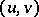by a position vector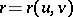that is continuous in the disc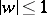,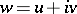, and that homeomorphically maps the circle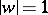onto; the area of this generalized minimal surface is the least among all continuous surfaces of disc type stretched on the contour, on the assumption that at least one such surface of finite area can be stretched on.

After the Plateau problem had been solved in 1931 for a simply-connected surface (J. Douglas), Douglas formulated the so-called Douglas problem on the existence in,, of a minimal surface having a given topological type (i.e. a given Euler characteristic and orientability character) and being bounded by a given contourconsisting of the union of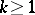Jordan curves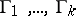. In 1936–1940, sufficient solvability conditions for this problem were given, one of which is the possibility of stretching some surface of a given topological type onwhose area is less than the area of any surface having a smaller Euler characteristic stretched on the same contour. In that formulation, the Plateau problem was considered and solved also in Riemannian spaces.

In the early 1960-s, a major advance was made in solving the Plateau problem for-dimensional surfaces,. Several generalizations of the Plateau problem were proposed, based on new definitions of the concept of a surface, a boundary and area. One of the extensions is based on the following definition of a surfaceand its boundaryin. Let there be a compact set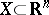, a compact set, letbe an Abelian group, and letbe an integer; then the Aleksandrov–Čech homology groups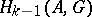,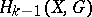are defined, together with the kernel of the homomorphisminduced by the imbedding, which is called the algebraic boundary of(in dimension) relative to. Ifis a subgroup of, thenis a surface with boundary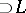ifbelongs to the algebraic boundary of; by the area of a compact setinone understands its-dimensional Hausdorff (spherical) measure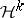. Existence and almost-everywhere regularity have been demonstrated for a compact set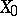that realizes the minimum in the measureover all compactawith the given boundarysubject to these stipulations (as well as topological local Euclidean structure and analyticity). Subsequently, these theorems were extended to the case of surfacesin a Riemannian space.

Other proposed generalizations, in particular in terms of integral currents, are in a certain sense equivalent to a formulation in homology terms.

The multi-dimensional Plateau problem (cf. Plateau problem, multi-dimensional) was solved in a classical formulation in 1969 (A.T. Fomenko), when the following theorem was proved: If one is given a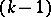-dimensional submanifold,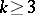, in a Riemannian space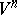, then there exists a surface that realizes a minimum in the Hausdorff measureamong all parametrized surfacesthat are continuous-transforms inof-dimensional smooth manifoldswith boundaries homeomorphic tounder the mapping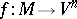.

Along with the solvability of the Plateau problem, interest attaches to uniqueness and regularity of the solution. Regularity has been most examined. It has been shown that the solution given by Douglas in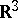does not contain interior branch points. Regularity almost everywhere has been demonstrated for the case of multi-dimensional Plateau problems, and the possibility of the existence of irregular points has been confirmed with examples. As regards uniqueness, only certain sufficient criteria are known (for example, the solution is unique if the given contourhas a single-valued convex projection under central or parallel projection onto a certain plane). To emphasise the complexity of this topic, it is sufficient to say that there is reason to expect the existence of smooth Jordan contours spanning a continuum of minimal surfaces of disc type. For a survey of recent results on the Plateau problem see .